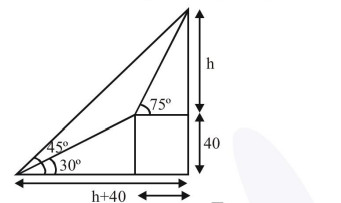# The angle of elevation of the top of a hill from

Question:

The angle of elevation of the top of a hill from a point on the horizontal plane passing through the foot of the hill is found to be $45^{\circ}$. After walking a distance of 80 meters towards the top, up a slope inclined at an angle of $30^{\circ}$ to the horizontal plane, the angle of elevation of the top of the hill becomes $75^{\circ}$. Then the height of the hill (in meters) is..

Solution:$\mathrm{h}+40-40 \sqrt{3}$

$\tan 75^{\circ}=\frac{h}{h+40-40 \sqrt{3}}$

$\frac{2+\sqrt{3}}{1}=\frac{\mathrm{h}}{\mathrm{h}+40-40 \sqrt{3}}$

$\Rightarrow 2 \mathrm{~h}+80-80 \sqrt{3}+\sqrt{3} \mathrm{~h}+40 \sqrt{3}-120=\mathrm{h}$

$\Rightarrow \mathrm{h}(\sqrt{3}+1)=40+40 \sqrt{3}$

$\Rightarrow \mathrm{h}=40$

$\therefore$ Height of hill $=40+40=80 \mathrm{~m}$